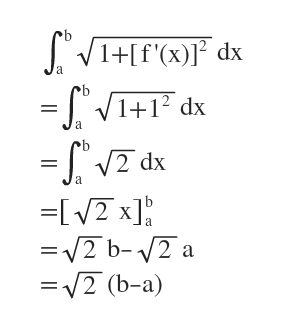# Show that if 0 ≤ f' (x) ≤ 1 for all x, then the arc length of y = f (x) over [a, b] is at most √2(b − a). Show that for f (x) = x, the arc length equals √2(b − a).

Question
1 views

Show that if 0 ≤ f' (x) ≤ 1 for all x, then the arc length of y = f (x) over [a, b] is at most √2(b − a). Show that for f (x) = x, the arc length equals √2(b − a).

check_circle

Step 1

The arc length increases with the value of f'(x)

Means, if f'(x) increases then arc length will increase too.

So, at f'(x)=1 the arc length will be maximum

Step 2

For f'(x)=1 arc length isThis is the maximum value of arc length.

Answer: If 0 ≤ f' (x) ≤ 1 for all x, then the arc length of y = f (x) over [a, b] is at most √2(b − a) [Proved]

...

### Want to see the full answer?

See Solution

#### Want to see this answer and more?

Solutions are written by subject experts who are available 24/7. Questions are typically answered within 1 hour.*

See Solution
*Response times may vary by subject and question.
Tagged in